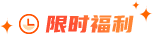### 生成所有的括号对题目描述

给定数字n，要求使用n对()括号生成所有合法的组合情况。

示例

3

[

"((()))",

"(()())",

"(())()",

"()(())",

"()()()"

]

题目解析

解法一： 暴力

暴力的解法应该最容易想到，因为n是确定的，也就是说一共有n个左括号和n个右括号。很容易想到，我们可以对这2n个字符进行排列组合，之后再对所有的组合进行过滤。留下合法的且不重复的即可。

伪代码很容易写：

def brute_force(str, l, r):

if l == n and r == n:

ans.append(str)

if l < n:

brute_force(str+'(', l+1. r)

if r < n:

brute_force(str+')', l, r+1)

写完了再根据结果判断是否合法，留下合法的所有情况即可。

这样编码的确不难，而且也很容易想到，但是计算n个字符的排列组合复杂度是 \$O(2^n)\$ 是一个指数级的算法，复杂度是我们不能接受的。而且根据上一题当中的结论，在匹配括号的时候是可以取巧的，我们其实没必要把所有的情况都枚举到。因为想要括号匹配合法，必须有一条，对于字符串当中的任何一个位置i，都必须有：前i个字符中所有左括号的数量大于等于右括号的数量。 ，否则就是非法的。

也就是说必须要保证任意一个位置右括号的数量小于等于左括号的数量，不然的话，多余的右括号永远也无法匹配。

解法二： 回溯

既然左括号的数量必须大于右括号的数量，我们完全可以据此进行优化。我们在递归的时候对l和r进行大小判断，保证所有时刻都有l >= r即可。

代码：

class Solution {

public:

void dfs(int n, int l, int r, string str, vector& vt) {

if (l+r == 2 *n) {

vt.push_back(str);

return ;

}

if (l < n) dfs(n, l+1. r, str+"(", vt);

if (r < l) dfs(n, l, r+1. str+")", vt);

}

vector generateParenthesis(int n) {

vector vt;

dfs(n, 0. 0. "", vt);

return vt;

}

};

解法三： 构造

这个方法是我原创的，官方的题解当中没有收录。

我们直接求解n的答案的时候是比较困难的，这个时候我们可以把问题拆解，大问题变成小问题，通过小问题的答案构造大问题的答案。上述的两种方法本质上也是一样的思路，不过递归替我们做了问题的拆分。

实际上我们可以自己拆分问题，n的时候我们一下子不清楚答案。我们可以先从简单的观察一下结果：比如当n1的时候，答案就是”()”。n2有两种：”()()”, “(())”。n==3的时候是5种：”((()))”, “()(())”, “()()()”, “(()())”, “(())()”。

细心的读者已经可以总结出规律了，其实并不难想到。

solution(n) = solution(i) + solution(n-i) + '(' solution(n-1) ')'

解释一下这个公式，这里的solution(n)表示n的所有答案串，也就是说n个括号的答案串是可以通过小于n的答案串进行组合的。比如n=1时答案是()，n=2则有两种，一种是用两个n=1拼接，第二种是在n=1的答案外层加上一个括号：

solution(2) = [()(), (())]

我们再来看solution(3)，它可以用n=1和n=2拼接，以及通过n=2外层加上单独的括号得到所有答案：

solution(3) = [()()(), ()(()), (())(), (()()), ((()))]

前面3种是通过solution(2)和solution(1)拼接得到的，后面两种则是在solution(2)外面直接加上括号得到的，这种情况是无法通过拼接得到的情况。我们把这些情况全部汇总，然后去除掉重复的情况就是答案了。

这样我们就用小于n的所有结果构造出了n的结果，由于n=0 和n=1的情况是已知的。我们只需要把中间结果存储下来，通过递推就可以获取所有的答案。

代码实现

class Solution {

public:

vector generateParenthesis(int n) {

// 存储所有中间结果

map> mp;

set st;

st.insert("()");

mp = st;

for (int i = 2; i <= n; i++) {

// 使用set来去重

set cur;

for (int j = 1; j <= i-1; j++) {

// 取出所有solution(j)和solution(i-j)

set vj = mp[j];

set vk = mp[i-j];

for (string str:vj) {

for (string stj : vk) {

cur.insert(str + stj);

}

}

}

// solution(i-1)最外层套上括号

set vj = mp[i-1];

for (string str : vj) {

cur.insert("(" + str + ")");

}

// 得到solution(i)

mp[i] = cur;

}

vector vt;

st = mp[n];

for (string str : st) vt.push_back(str);

return vt;

}

};### 大数据师资团队更多>>

• 北京校区
• 大连校区
• 广州校区
• 成都校区
• 杭州校区
• 长沙校区
• 合肥校区
• 南京校区
• 上海校区
• 深圳校区
• 武汉校区
• 郑州校区
• 西安校区
• 青岛校区
• 重庆校区
• 太原校区
• 沈阳校区
• 南昌校区
• 哈尔滨校区